# Why won't my event seem to work?

#### unmerged(47151)

##### Colonel
Code:
``````rovince_event = { # Peasants take power ending revolt
id = 40109

picture = "event_prosperity"

trigger = {
condition = { type = has_province_effect value = { revolt = yes } }
[COLOR="Red"]		condition = {
type = not
value = { type = peasant_loyalty value = 0.5 }
}[/COLOR]
condition = { type = owner
condition = { type = trait value = user_defined_b }
condition = { type = trait value = merciful }
condition = { type = has_law value = { royal_preorgatory_law = yes } }
condition = { type = has_law value = { elective_law = yes } }
}
}

mean_time_to_happen = {
months = 1

}

action_a = { # Mild Reforms
ai_chance = 25
effect = { type = remove_province_effect value = revolt }
effect = { type = peasant_loyalty value = 0.2 }
effect = { type = peasant_power value = 0.1 }
effect = { type = owner
effect = { type = add_trait value = wise }
effect = { type = add_trait value = forgiving }

}

action_b = { # Radical Reforms
ai_chance = 50
effect = { type = remove_province_effect value = revolt }
effect = { type = peasant_loyalty value = 0.4 }
effect = { type = peasant_power value = 0.2 }
effect = { type = owner
effect = { type = add_trait value = just }
effect = { type = add_trait value = vengeful }

}

action_c = { # Social Revolution
ai_chance = 20
effect = { type = remove_province_effect value = revolt }
effect = { type = peasant_loyalty value = 0.4 }
effect = { type = peasant_power value = 0.2 }
effect = { type = owner
effect = { type = add_trait value = reckless }
effect = { type = add_trait value = vengeful }
}

action_d = { # Crush Them!
ai_chance = 5
effect = { type = peasant_loyalty value = -0.4 }
effect = { type = owner
effect = { type = add_trait value = cruel }
effect = { type = add_trait value = deceitful }
}
}``````

I've been progressing well in my MOD but for some reason this event seems not to work. The game complains that the event outlined in red has wrong ihs, but I've already tried an earlier more usual arrangement and that didn't work. That is the setup the event generator gives me for the peasant_loyalty condition.

So what is wrong with my event? Anyone know?

#### Veldmaarschalk

##### Very old guard
Super Moderator
Moderator
•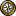•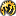•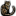•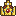•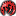I can't see anything wrong with it, but have you tried the CK Validator ?

Since the error means you are missing a { or }.

#### unmerged(47151)

##### Colonel
I can't see anything wrong with it, but have you tried the CK Validator ?

Since the error means you are missing a { or }.

The validator complains that
Code:
``````--- Error 1 of 1 ---
Parse Failure
Error: Token "{" at line 245, column 35 must be an equals sign.``````

This is line 245.

Code:
``condition = { type = not value = { type = peasant_loyalty value = 0.5 } }``

Apparantly it wants column 35, which I take must mean the following letter.

Code:
``condition = { type = not value = [COLOR="Red"]{[/COLOR] type = peasant_loyalty value = 0.5 } }``

I take it wants me to delete the { and also presumably the type = and have only a = sign.

#### unmerged(47151)

##### Colonel
No removing the { doesn't work. I take it wants me to layer it in some way. The exact same command worked before here.
Code:
``````character_event = { # Establish nature of rebellion as peasants
id = 40108

picture = "event_rebellion"

trigger = {
condition = { type = ruler }
condition = { type = trait value = user_defined_b }
condition = { type = has_law value = { traditional_custom_law = yes } }
condition = { type = has_law value = { semisalic_primogeniture_law = yes } }
condition = { type = has_law value = { ecclesical_balance = yes } }
condition = { type = capital
condition = { type = has_province_effect value = { revolt = yes } }
[COLOR="Green"]			condition = { type = not value { type = peasant_loyalty value = 0.5 } }[/COLOR]
}
}

mean_time_to_happen = {
months = 1

}

action_a = {
effect = { type = set_law value = royal_preorgatory_law }
effect = { type = set_law value = elective_law }
effect = { type = add_trait value = merciful }
effect = { type = remove_trait value = deceitful }
effect = { type = remove_trait value = honest }
effect = { type = remove_trait value = vengeful }
effect = { type = remove_trait value = forgiving }
effect = { type = remove_trait value = wise }

}
}``````

How would I go about writing such a condition so that it would work?

#### unmerged(410089)

##### Recruit
Hi Guys,

It's my first time actually working with the event psuedo codes and the syntax is a bit different from normal programming but I'm familiar with coding in general so here's my feedback.

From a basic open { and close }, the top part looks fine, but you're missing the closing } on all action_a, action_b, action_c and action_d for the type = owner clauses. I'm wondering if that might be throwing the exception errors?

All the best.

#### unmerged(47151)

##### Colonel
Code:
``````province_event = { # Peasants take power ending revolt
id = 40109

picture = "event_prosperity"

trigger = {
condition = { type = has_province_effect value = { revolt = yes } }
condition = { type = not value = type = peasant_loyalty value = 0.5 }
condition = { type = owner
condition = { type = trait value = user_defined_b }
condition = { type = trait value = merciful }
condition = { type = has_law value = { royal_preorgatory_law = yes } }
condition = { type = has_law value = { elective_law = yes } }
}
}``````

Apparantly you have to use a double = with no brackets. This was tricky because the exact same problem also existed somewhere else in the file, causing massive frustration.